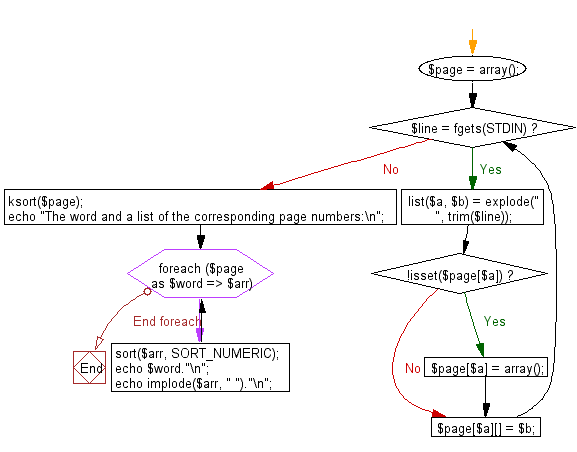﻿ PHP Exercise: Reads a list of pairs of a word and a page number, and prints the word and a list of the corresponding page numbers - w3resource

# PHP Exercises: Reads a list of pairs of a word and a page number, and prints the word and a list of the corresponding page numbers

## PHP: Exercise-77 with Solution

Write a PHP program which reads a list of pairs of a word and a page number, and prints the word and a list of the corresponding page numbers.
The number of pairs of a word and a page number is less than or equal to 1000. A word never appear in a page more than once. The words should be printed in alphabetical order and the page numbers should be printed in ascending order.

Sample Output:
The maximum value of the sum of integers passing according to the rule on one line.

Sample Solution: -

PHP Code:

``````<?php
\$page = array();
while(\$line = fgets(STDIN)){
list(\$a, \$b) = explode(" ", trim(\$line));
if(!isset(\$page[\$a])){
\$page[\$a] = array();
}
\$page[\$a][] = \$b;
}
ksort(\$page);
echo "The word and a list of the corresponding page numbers:\n";
foreach(\$page as \$word => \$arr){
sort(\$arr, SORT_NUMERIC);
echo \$word."\n";
echo implode(\$arr, " ")."\n";
}

?>
```
```

Sample Input:
apple 5
banana 6

Sample Output:

```The word and a list of the corresponding page numbers:
apple
5
banana
6```

Flowchart:PHP Code Editor:

Have another way to solve this solution? Contribute your code (and comments) through Disqus.

What is the difficulty level of this exercise?

Test your Programming skills with w3resource's quiz.

﻿

## PHP: Tips of the Day

Mutates the original array to filter out the values specified

Example:

```<?php
function tips_pull(&\$items, ...\$params)
{
\$items = array_values(array_diff(\$items, \$params));
return \$items;
}

\$items = ['x', 'y', 'z', 'x', 'y', 'z'];
print_r(tips_pull(\$items, 'y', 'z'));
?>
```

Output:

```Array
(
 => x
 => x
)
```